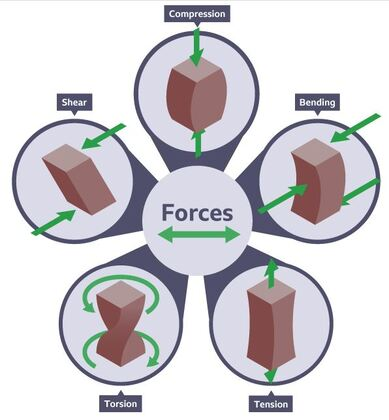# Types of Forces and Stresses

Last Updated on July 18, 2022

## What is Force?

In order to understand the types of forces and stresses in civil engineering, let’s start with the terms force and stress. A push or pull that an object experiences as a result of interacting with another object is known as a force. Every time two objects interact, a force is exerted on each of the objects. The force is no longer felt by the two objects when the interaction finishes. Only when there is interaction or induction between two objects, forces by the objects actually exist.

When an object pushes or pulls on another, the former object applies a force to the other object. As an example, a man pushing a door to open is applying force to the door. Similar to this, a girl pulling a bag by its handle exerts force on the handle.

## What are action at a distance forces?

Action at a distance forces are those that occur even when the two objects are not physically touching each other but are still able to push or pull against each other despite their physical separation. Gravitational forces are an example of action at a distance forces. For instance, despite their great distance from one another in space, the sun and planets still pull on each other through gravity. There is a gravitational pull between you and the Earth even after your feet and you have lost all physical contact with it.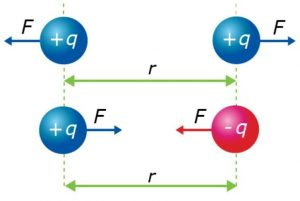Electric forces are forces that act at a distance. For instance, despite their slight spatial difference, the protons in the atom’s nucleus and the electrons outside the nucleus feel an electrical pull against each other. Additionally, magnetic forces are forces that act at a distance. To  illustrate, two magnets can still pull on each other magnetically even though they are a few centimeters apart.

## What is Stress in Civil Engineering?

Stress is defined as “force per unit area” and is the product of the applied force F (or P) over the cross-sectional area. In a much more general sense, the concept of stress might be used to understand the situation at any point inside a solid. Stress could also be utilized to estimate when a material will fail.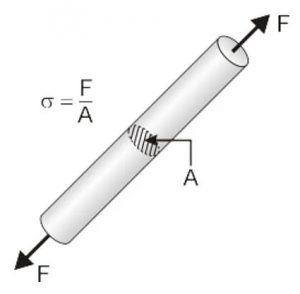In other words, when a force is applied to a specific cross-sectional area of an object, the loading is expressed in terms of stress (σ). Stress is the applied force or system of forces that tends to deform a body from the viewpoint of loading. Also, stress is the internal distribution of forces within a body that resist and balance to the loads applied to it from the viewpoint of what is happening inside the material. Depending on the type of loading, the stress distribution might or might not be uniform. For instance, a uniform bar loaded in tension axially will basically have a uniform distribution of tensile stress. A bar loaded for bending, however, will have a stress distribution that varies with the distance from the normal axis.

### What is the stress formula?

Stress formula is written as:

σ=P/A

Where σ = stress (Pa), P = force (N) and A = cross-sectional area (m2)

Newton/m2 = Pascal

We may say that there are mainly 5 types of forces and stresses in civil engineering which are tensile forces, compressive forces, flexural stress, shear forces and torsion. Force is described as an action that causes or tends to cause a change in the state of rest or motion of a body.
The unit of force is Newton (N).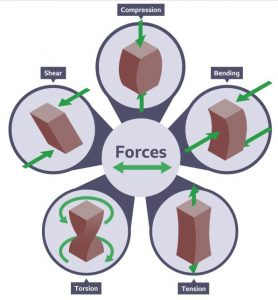## Main Types of Forces and Stresses in Civil Engineering

### 1. Tensile Force

The stretching forces acting on the material are referred to as tensile force, which has two components: tensile stress and tensile strain. This indicates that the material being acted upon is under tension, and that the forces are attempting to stretch it. When a tensile force is applied to a material, it produces a stress corresponding to the applied force, causing the cross-section to become thinner and the length of the material to longer• Stress is calculated as: Stress (σ) = Tensile force (F)/Area (A)
• Strain is calculated as: Strain (ε) = Amount of elongation (ΔL)/Original Length (L)

### 2. Compressive Force

When a force presses inward on a material, forcing it to compact, it is called as a compressive force or compression force. The relative positions of the material’s atoms and molecules change through this process. Depending on the form of material experiencing the compressive force, this effect may be temporary or permanent. Different outcomes can be obtained depending on the direction or position of the compressive force applied to the material.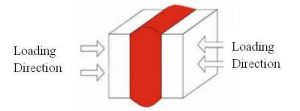Compressive stress formula:

CS=F/A where;

F=force

A=area

In other words, a material’s compression strength refers to its ability to resist external types of forces. A column is under compressive stress as it carries a load from above. On a molecular basis, this force causes the particles, atoms and molecules in the material, to shorten.

Since loads and pressures must inevitably be directed into the earth, compression is a factor in all structures. It’s also a principle used in engineering when materials like gravel and rocks have to be forced into a stable, compacted state. Building codes typically require material under slabs, concrete, or other foundation surfaces to have at least 90 percent compaction rating; testing laboratories determine the degree to which material under a building has to be compacted.

### 3. Shear Force

Shear forces are types of forces that acts perpendicular to a surface and opposes an offset force that acts in the opposite direction. Shear stress is the result of this. To put it another way, one section of the surface is forced in one direction while another is forced in the opposite direction.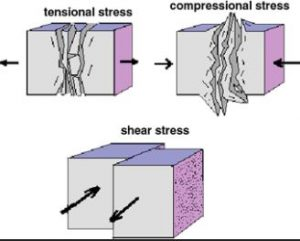Shear stress formula:

τ=F/A where;

τ=shear stress

F=the force applied

A=the cross-sectional area of the material that is perpendicular to applied force.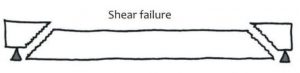When a structural member fails by shear, two parts of it are pulled in opposite directions, similar to how a sheet of paper is cut with scissors. Shear walls are used in large or high-rise buildings to withstand shear forces that may cause parallel structural elements of the building fail, causing racking.

### 4.Flexural Strength (Bending Strength)

The maximum bending stress that can be applied to a material just before it yields is known as its flexural strength. A transverse bending test using a three-point flexural test technique is the most common method of determining a material’s flexural strength.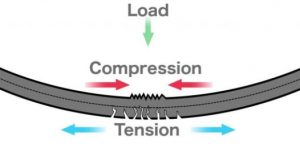For a three-point test, the flexural strength (σ) can be calculated as:

σ = 3FL / 2wd2

where  σ=flexural strength

F=maximum applied force

L=length of specimen

d=depth of specimen

w=width of specimen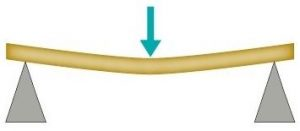When a specimen is bent, typically a beam or rod, it is subjected to a range of stresses in its depth. The stress would be at its highest compressive stress on the inside of the bend, and its highest tensile stress on the opposite side.

Extreme fibres refer to the inner and outer edges of the specimen. Tensile stress causes most materials to fail before compressive stress failure. Small defects of different sizes on the surface cause this, and they can increase in size under tensile stress.

### 5. Torsion

The condition of tension in a material that has been bent by an applied torque is known as torsion. When a structural element is subjected to a bending motion, this occurs. A circular-section rubber bar inscribed with rectangles and held at each end with one hand rotating opposite to the other demonstrates torsion: the rectangles become bent – or twisted. Torsion is the state of tension that has distorted the rectangles, and it is comprised of pure shear. The torsion in the rubber bar would attempt to return it to its original shape.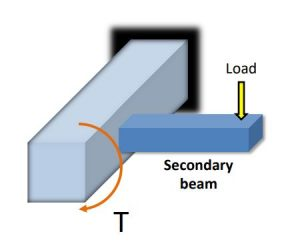The rigidity of the member cross-section determines how well it can withstand torsional shear stress. As a result, torsion should be considered when designing edge beams. Vertical members like columns and shear walls should be designed to withstand complex loads like earthquakes and wind.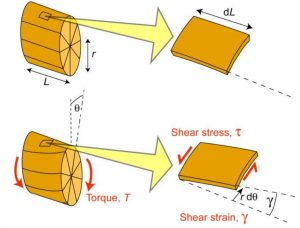Hooke’s law applies to linear-elastic materials.
• Along any radial line on the cross section, a linear difference of shear strain causes a linear variation in shear stress.
To calculate the shear stress:

τ max = T*c/J or τ=T*p/J

where τ=shear stress

T=resultant internal torque

J=polar moment of inertia of cross-sectional area

p=intermediate distance

### 6. Punching Force (Punching Shear)

Punching resembles shearing in several ways. When you press the tip of a pen on a sheet of paper, the force applied by the pen tip on the paper is known as punching force. This punching force will attempt to pierce the page. Similarly, pillars and columns apply force to the slab and foundation. Punching can be adjusted by increasing the thickness of the material and increasing its strength.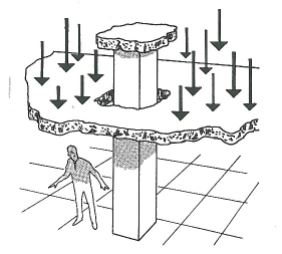In other words, punching shear forces are types of forces that is very similar to shear failure process that occurs in structural members such as slabs and foundations when concentrated loads are applied. Concentrated loads act on a small surface area in structural members. This reaction is usually caused by the column working against the slab. The load punching through the slab is one potential form of failure.

### 7. Frictional Force

Friction is the resistance to movement that occurs as two surfaces roll or slide over one another; one surface can be stationary while the other is moving. The degree of friction in an engineering sense usually reflects the energy lost, typically as heat, and is thus minimized. This may include things like construction, material choice, coatings or surface treatments, as well as the use of lubricating fluids or solids.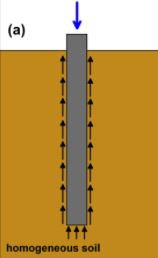Friction forces are types of forces which become very useful in piled foundations. The frictional resistance force between the pile surface and the adjacent soil is used to transfer the superstructure load to ground. Resistance force due to friction will build in a definite pile length or on the maximum length depending on the subsoil strata condition. The friction force must be sufficient to maintain the superstructure for a stable foundation state.

Types of forces and stresses in civil engineering have been explained in this article. Hope you enjoyed it!